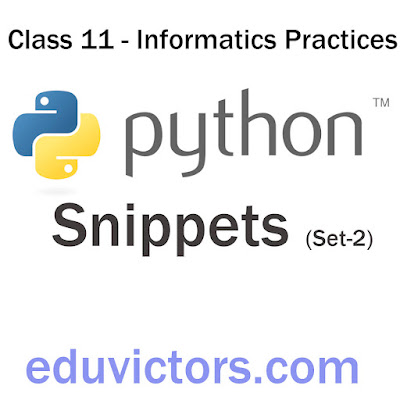# Class 11 - Informatics Practices - Python Snippets (Set-2)

Write Python Programs for the following problem statements:

1. Write a program in python to input marks in 5 subjects from the user and display the average marks.

2. Write a program in python to read details like name, class, age of a student and then print the details firstly in same line and then in separate lines.

3. Write a program in python to input a number and print its first five multiples.

4. Write a program to read a number n and print n, n² , n³ and n⁴

5. Write a program that generates the following output: 5 @ 10 @ 9

Snippet 1: Write a program in python to input marks in 5 subjects from the user and display the average marks.

```# Write a program in python to input marks in 5 subjects
# from the user and display the average marks.

print("Enter marks out of 50 for the following subjects:")
sub1 = int(input("Maths:"))
sub2 = int(input("English:"))
sub3 = int(input("Pol Science:"))
sub4 = int(input("Geography:"))
sub5 = int(input("Informatics:"))
avg = (sub1 + sub2 + sub3 + sub4 + sub5)/5

```

Program output:

Snippet 2
Write a program in python to read details like name, class, age of a student and then print the details firstly in same line and then in separate lines.

```# python snippet 2 by eduvictors.com
# Write a program in python to read details like name,
# class, age of a student and then print the details firstly in
# same line and then in separate lines.

stud_name = input("Enter you name:")
stud_age = int(input("Enter your age in years:"))

print(stud_name, stud_class, stud_age)
print("Student Name:", stud_name)
print("Student Class:", stud_class)
print("Student Age:", stud_age)
```

Output:

```# Snippet 3 by eduvictors.com
# Write a program in python to input a number and print
# its first five multiples.

n = int(input("Enter an integer: "))
print("First five multiples of", n, "are")
print(n, n * 2, n * 3, n * 4, n * 5)
```

Output:

Snippet 4: Write a program to read a number n and print n, n² , n³ and n⁴

```# Snippet 4 by eduvictors.com
# Write a program to read a number n and print n, n² , n³ and n⁴

n = int(input("Enter an integer: "))
print(n, n ** 2, n ** 3, n ** 4)
```

Output:

Snippet 5Write a program that generates the following output: 5 @ 10 @ 9

```# Snippet 5 by eduvictors.com
# Write a program that generates the following output:
# 5 @ 10 @9

n = 5
print(n, n * 2, n * 2 - 1, sep=' @ ')
```

Output: